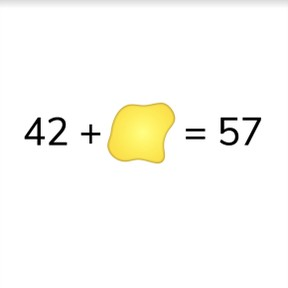Fill in the blank problems + - to 100

# Fill in the blank problems + - to 100

Solve fill in the blank math problems with addition & subtraction with numbers t8,000 schools use Gynzy92,000 teachers use Gynzy1,600,000 students use Gynzy

## General

Students learn to add and subtract in fill in the blank math problems up to 100.

## Standards

CCSS.Math.Content.2.NBT.B.5

## Learning objective

Students will be able to add and subtract up to 100 in fill in the blank problems.

## Introduction

The interactive whiteboard shows five fish with numbers and five fishbowls with numbers. The students must match the fish to the fishbowl that has a total of one hundred. Draw a line from the fish to the fishbowl.

## Instruction

Explain that fill in the blank problems are math problems in which one part of the math problem is missing. Tell students that you will first discuss how to solve fill in the blank addition problems, and then fill in the blank subtraction problems. Explain to students that when trying to determine the blank in an addition problem you can take away the number you have from the total. For example- if you take the addition problem 27 + ... = 81 you make the subtraction problem 81 - 27 = 54. Show this again using an addition problem in which the second addend is blank. Next explain to students that when solving a fill in the blank subtraction problem where the second number (the subtrahend) is missing, you can easily take the first number (the minuend) from the difference to determine the second number (the subtrahend). For example- if you take the subtraction problem 87 - ... = 63 you turn it into the subtraction problem 87 - 63 = 24. If you need to determine what the first number (the minuend) of a subtraction problem is in a fill in the blank problem, explain to students that you can add the second number (the subtrahend) and the difference together to determine the first number (the minuend). Show this using ... - 52 = 19, and turn it into 52 + 19 = 71. The interactive whiteboard then shows four fill in the blank problems. The students are given four math problems that represent how to determine the missing number. Ask students to drag the correct math problems to the thought bubbles. The students are then given fill in the blank problems as well as numbers that complete the given math problems. Ask students to drag the numbers to the blanks and have students explain their strategy as they do so. Finally there are a few problems given in which the plus or minus sign is missing. Ask students to determine which one the math problem is missing. You can check their answers by erasing the grey square.

Check that students are able to solve fill in the blank math problems with addition & subtraction with numbers to 100 by asking the following questions:
- How can you easily determine which number belongs in the empty space of a math problem?
- What kind of math problem do you make to solve 65 + ... = 82?
- What kind of math problem do you make to solve ... - 27 = 51?

## Quiz

Students are first asked to fill in the blank with addition problems. Then they are given subtraction problems. They are also asked to determine which answer correctly completes the problem with either a plus or minus sign or plus/minus sign and a number.

## Closing

Remind students that it is important to be able to determine which number fills in the blank and ask students how they can figure out which number completes a given math problem. The interactive whiteboard then has a collection of math problems which are your homework, but your dog walked all over and left muddy paw prints! Ask students to figure out which numbers complete the given math problems. You may have them work individually or in pairs. Make sure to have them explain their thinking. You can "clean off" the paw prints to reveal the answers.

## Teaching tips

Students who have difficulty with this learning goal can be supported by making use of a number line. They can count in steps from the given number to the total or to the difference.

### The online teaching platform for interactive whiteboards and displays in schools

• Save time building lessons

• Manage the classroom more efficiently

• Increase student engagement